# Large sieve

(diff) ← Older revision | Latest revision (diff) | Newer revision → (diff)

A method developed by Yu.B. Linnik in 1941 which permits one to sift out in sequences of increasing numbers the residues to be discarded. Its essence may be explained as follows. Consider a given sequence of positive integers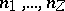not larger than, a prime number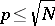and a residue,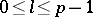. Let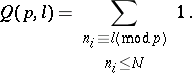It follows from statistical considerations, which may be rigorously proved with the aid of the fundamental idea of the circle method, that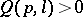for almost-all, and hence also for almost-all. Letbe the number of such's, and let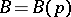be the number of corresponding's. Linnik showed that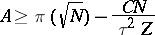andwhere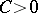is a constant and, and deduced the theorem: The number of primes on the segment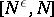to which Vinogradov's hypothesis on the least square non-residue (cf. Vinogradov hypotheses) does not apply can only be finite (depending on).

There exists an improved method of the large sieve in which the average values of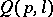are estimated. The best result is due to E. Bombieri (1965):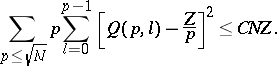The method of the large sieve made its most important contribution to modern analytic number theory in the context of the density method; this resulted in a proof of the Vinogradov–Bombieri theorem (1965) — the averaged asymptotic law of prime numbers in progressions. This and other similar theorems about the average found extensive application in the solution of several familiar problems in number theory, replacing the generalized Riemann hypothesis (cf. Riemann hypothesis, generalized) in many cases.

How to Cite This Entry:
Large sieve. Encyclopedia of Mathematics. URL: http://encyclopediaofmath.org/index.php?title=Large_sieve&oldid=17626
This article was adapted from an original article by B.M. Bredikhin (originator), which appeared in Encyclopedia of Mathematics - ISBN 1402006098. See original article